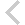FICO Xpress Optimization Examples Repository
 FICO Optimization Community FICO Xpress Optimization HomeIntroductory examples

Description
approx burglar chess Problem name and type, features Difficulty Approximation: Piecewise linear approximation ** SOS-2, Special Ordered Sets, piecewise linear approximation of a nonlinear function, pwlin MIP modeling: Knapsack problem: 'Burglar' * simple MIP model with binary variables, data input from text data file, array initialization, numerical indices, string indices, record data structure LP modeling: Production planning: 'Chess' problem * simple LP model, solution output, primal solution values, slack values, activity values, dual solution values All item discount pricing: Piecewise linear function *** SOS-1, Special Ordered Sets, piecewise linear function, approximation of non-continuous function, step function, pwlin Incremental pricebreaks: Piecewise linear function *** SOS-2, Special Ordered Sets, piecewise linear function, step function

Further explanation of this example: 'Applications of optimization with Xpress-MP', Introductory examples (Chapters 1 to 5) of the book 'Applications of optimization with Xpress-MP'

Source Files
By clicking on a file name, a preview is opened at the bottom of this page.

Data Files

approx.mos

(!******************************************************
Mosel Example Problems
======================

file approx.mos

Function approximation with SOS2

SOS2s are generally used for modeling piecewise approximations
of functions of a single variable. In this example, we aim
to represent f as a function of x and we consider four line
segments between five points. The five points are (R1, F1),
(R2, F2), (R3, F3), (R4, F4) and (R5, F5) and associated with
each point i is a weight variable y(i). Binary variable b(i) is
associated with each of the intervals (R1, R2), (R2, R3), (R3,
R4) and (R4, R5), so 'b(i)' takes value 1 if the value of x
lies between 'R(i)' and 'R(i+1)'.

The SOS2 property of having at most two non-zero 'y(i)', and
if there are two non-zero then they must be adjacent, implies
that we are always on the piece-wise linear function.

(c) 2008 Fair Isaac Corporation
author: S. Heipcke, Sep. 2006
*******************************************************!)

model "Approximation"
uses "mmxprs"

declarations
NB = 5
BREAKS = 1..NB
R,F: array(BREAKS) of real           ! Coordinates of break points
x,f: mpvar                           ! Decision variables
y: array(BREAKS) of mpvar            ! Weight variables
end-declarations

R:: [1, 2.2, 3.4, 4.8, 6.5]
F:: [2, 3.2, 1.4, 2.5, 0.8]

RefRow:= sum(i in BREAKS) R(i)*y(i)
x = RefRow
f = sum(i in BREAKS) F(i)*y(i)

! Convexity constraint
sum(i in BREAKS) y(i) = 1

! SOS2 definition
RefRow is_sos2

! Alternative SOS definition (to be used for 0-valued RefRow coefficients):
! makesos2(union(i in BREAKS) {y(i)}, RefRow)

(! Alternative formulation using binaries instead of SOS2:
declarations
b: array(1..NB-1) of mpvar
end-declarations

sum(i in 1..NB-1) b(i) = 1
forall(i in 1..NB-1) b(i) is_binary
forall(i in BREAKS) y(i) <= if(i>1, b(i-1), 0) + if(i<NB, b(i), 0)
!)

! Bounds
1<=x; x<=6.5

! Solve the problem
minimize(f)

writeln("Objective value: ", getobjval)
writeln("x: ", getsol(x))

end-model

`